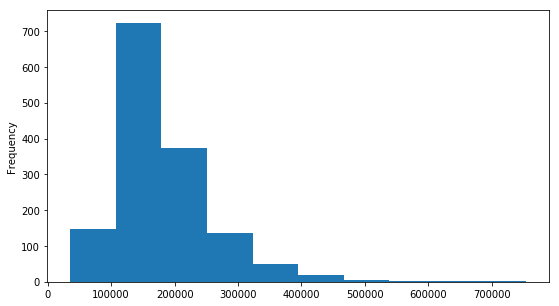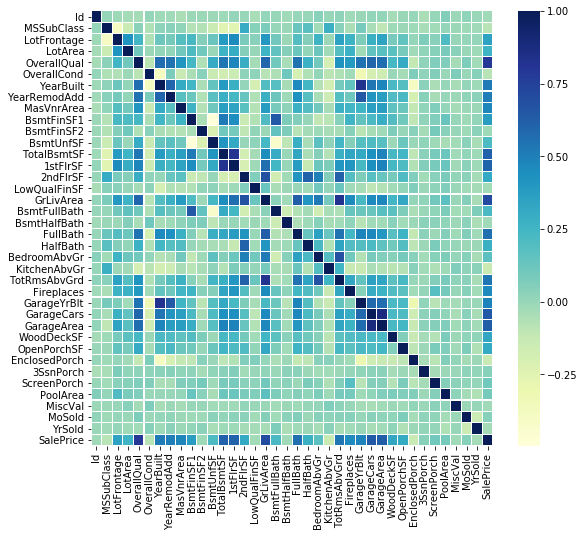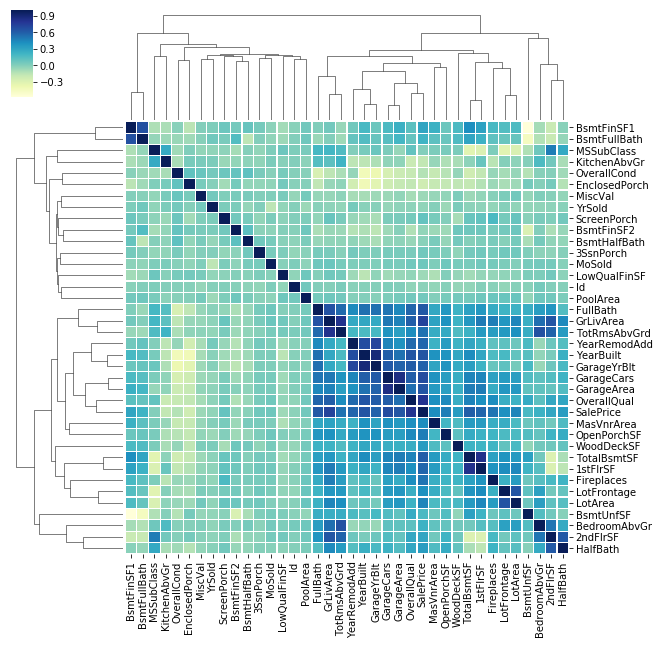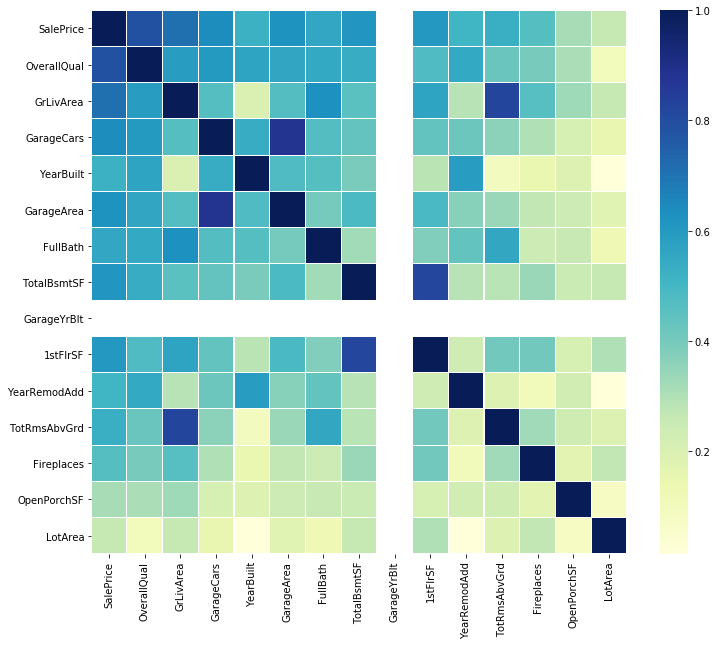# Exploring Correlation in Python

This article aims to give a better understanding of a very important technique of multivariate exploration.

Correlation Matrix is basically a covariance matrix. Also known as the auto-covariance matrix, dispersion matrix, variance matrix, or variance-covariance matrix. It is a matrix in which i-j position defines the correlation between the ith and jth parameter of the given data-set.

When the data points follow a roughly straight-line trend, the variables are said to have an approximately linear relationship. In some cases, the data points fall close to a straight line, but more often there is quite a bit of variability of the points around the straight-line trend. A summary measure called the correlation describes the strength of the linear association. Correlation summarizes the strength and direction of the linear (straight-line) association between two quantitative variables. Denoted by r, it takes values between -1 and +1. A positive value for r indicates a positive association, and a negative value for r indicates a negative association.
The closer r is to 1 the closer the data points fall to a straight line, thus, the linear association is stronger. The closer r is to 0, making the linear association weaker.

 `import` `numpy as np ` `import` `pandas as pd ` `import` `seaborn as sns ` `import` `matplotlib.pyplot as plt ` `from` `scipy.stats ``import` `norm `

 `data ``=` `pd.read_csv(``"House Price.csv"``) ` `data.shape `

Output:

`(1460, 81)`

‘Sales Price’ Description

 `data[``'SalePrice'``].describe() `

Output:

```count      1460.000000
mean     180921.195890
std       79442.502883
min       34900.000000
25%      129975.000000
50%      163000.000000
75%      214000.000000
max      755000.000000
Name: SalePrice, dtype: float64```

Histogram

 `plt.figure(figsize ``=` `(``9``, ``5``)) ` `data[``'SalePrice'``].plot(kind ``=``"hist"``) `

Output:Code #1: Correlation Matrix

 `corrmat ``=` `data.corr() ` ` `  `f, ax ``=` `plt.subplots(figsize ``=``(``9``, ``8``)) ` `sns.heatmap(corrmat, ax ``=` `ax, cmap ``=``"YlGnBu"``, linewidths ``=` `0.1``) `

Output:Code #2: Grid Correlation Matrix

 `corrmat ``=` `data.corr() ` ` `  `cg ``=` `sns.clustermap(corrmat, cmap ``=``"YlGnBu"``, linewidths ``=` `0.1``); ` `plt.setp(cg.ax_heatmap.yaxis.get_majorticklabels(), rotation ``=` `0``) ` ` `  `cg `

Output:Code #3: Correlation for Saleprice

 `# saleprice correlation matrix ` `# k : number of variables for heatmap ` `k ``=` `15`  ` `  `cols ``=` `corrmat.nlargest(k, ``'SalePrice'``)[``'SalePrice'``].index ` ` `  `cm ``=` `np.corrcoef(data[cols].values.T) ` `f, ax ``=` `plt.subplots(figsize ``=``(``12``, ``10``)) ` ` `  `sns.heatmap(cm, ax ``=` `ax, cmap ``=``"YlGnBu"``, ` `            ``linewidths ``=` `0.1``, yticklabels ``=` `cols.values,  ` `                              ``xticklabels ``=` `cols.values) `

Output:My Personal Notes arrow_drop_upIf you like GeeksforGeeks and would like to contribute, you can also write an article using contribute.geeksforgeeks.org or mail your article to contribute@geeksforgeeks.org. See your article appearing on the GeeksforGeeks main page and help other Geeks.

Please Improve this article if you find anything incorrect by clicking on the "Improve Article" button below.